Start typing, then use the up and down arrows to select an option from the list.# Osmolarity Example 3

Jules Bruno
118views
1
Method three involves the number of ions from polarity. Now we're going to say here problems involving number of ions and polarity can use a given amount and converting factors to isolate and end amount. Now, for those of you who haven't watched my videos on dimensional analysis and conversion factors, these terms basically formed the foundations for a lot of the calculations you'll see in chemistry. Your conversion factor is basically just when you have two different units connected together that is seen in polarity. Because morality itself represents moles per liter, two units connected together are given amount is just a value that's given to us. That has only one unit that would be represented by these leaders here and then Our end amount is just simply the value or the amount that we're trying to find at the end. Now, if we look at this question, remember, when we have the word off in between numbers, it means that we have to multiply them together. So we're going to say here are given amount. Where we're starting is 0.1 to 0 leaders, and we're gonna have to utilize conversion factors to get to our end amount our end amount we're looking for is moles of calcium ions. Now we need to cancel out leaders here. We're able to do that by utilizing the polarity given to us. The polarity is 0.450 Mueller, which means 0.450 moles of calcium phosphate per one, leader of solution. So that's our first conversion factor. Then leaders cancel out. Now we have to just change moles of calcium phosphate into moles of just calcium ion. So here, for every one mole of our entire compound, we can see that from the formula. There's a little three there, which means that I have three moles of calcium ions. So then, if we multiply everything out that's on top, I'll be left with 0.162 moles off calcium ion as my final answer.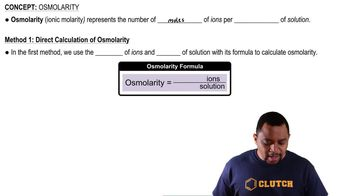01:10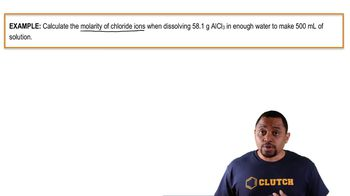02:55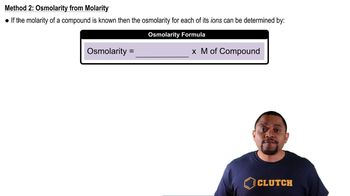00:37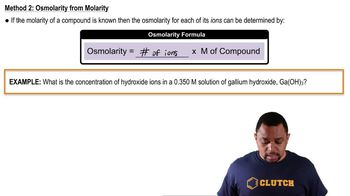01:16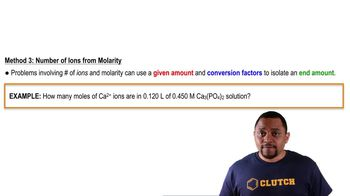02:18